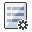The Rocscience International Conference 2021 Proceedings are now available. Read Now

# Slope Properties

If you are performing a Probabilistic analysis with SWedge, the following slope properties can be defined as random variables:

• Slope Face orientation (dip and dip direction)
• Upper Face orientation (dip and dip direction)

To define the slope plane orientations as random variables:

1. Select Input Dataand select the Slope or Upper Face tab.
2. Select a statistical distribution for the Dip and/or Dip Direction.
3. Enter the mean orientation values and statistical parameters.

## Constant Slope Properties

The following slope parameters CANNOT be defined as random variables for a Probabilistic analysis (i.e. only a single constant value can be defined for each parameter, for a given probabilistic simulation):

However, it is worth noting that the Slope Height, Rock Unit Weight, Bench Width and Slope Length can be defined as variables for a Sensitivity Analysis, if the Analysis Type = Deterministic.